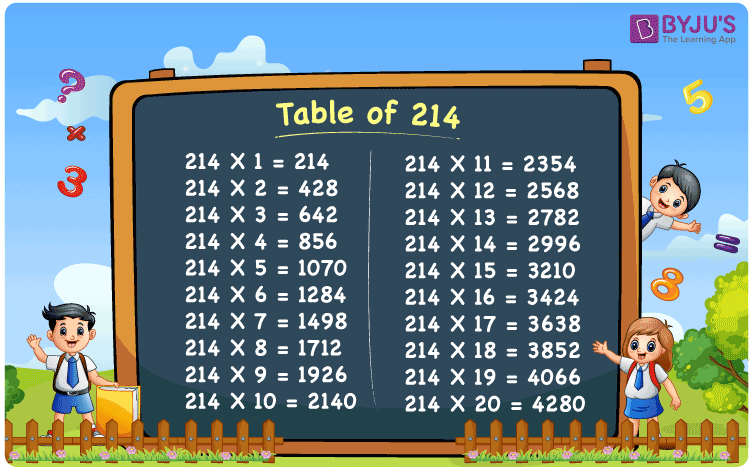Checkout JEE MAINS 2022 Question Paper Analysis : Checkout JEE MAINS 2022 Question Paper Analysis :

# Table of 214

The Table of 214 is created by the experts at BYJU’S in order to help students ace the exams, irrespective of their intelligence quotient. Once multiplication tables are mastered, students will become more knowledgeable in their day to day life. The PDF format given here contains multiplication of 214 with number till 20. An example of repeated addition is 214 + 214 + 214 + 214 = 856, but from the multiplication table, 214 x 4 = 856. The PDF of the multiplication table of 214 can be referred by the students by just clicking on the link given below.

## Table of 214 Chart## What is the 214 Times Table?

The multiplication table of 214 is designed with utmost care by the faculty at BYJU’S after conducting a vast research on the basic concepts. The PDF of multiplication tables is absolutely free of cost in order to improve the problem solving abilities among the students from their primary levels of education itself.

 214 × 1 = 214 214 214 × 2 = 428 214 + 214 = 428 214 × 3 = 642 214 + 214 + 214 = 642 214 × 4 = 856 214 + 214 + 214 + 214 = 856 214 × 5 = 1070 214 + 214 + 214 + 214 + 214 = 1070 214 × 6 = 1284 214 + 214 + 214 + 214 + 214 + 214 = 1284 214 × 7 = 1498 214 + 214 + 214 + 214 + 214 + 214 + 214 = 1498 214 × 8 = 1712 214 + 214 + 214 + 214 + 214 + 214 + 214 + 214 = 1712 214 × 9 = 1926 214 + 214 + 214 + 214 + 214 + 214 + 214 + 214 + 214 = 1926 214 × 10 = 2140 214 + 214 + 214 + 214 + 214 + 214 + 214 + 214 + 214 + 214 = 2140

## Multiplication Table of 214

The Table of 214 has to be memorized by the students in order to speed their calculation in the final exams. The time duration given in competitive exams will be less, for this reason, learning all the tables by heart will help you score good rank and achieve your career goals.

 214 × 1 = 214 214 × 2 = 428 214 × 3 = 642 214 × 4 = 856 214 × 5 = 1070 214 × 6 = 1284 214 × 7 = 1498 214 × 8 = 1712 214 × 9 = 1926 214 × 10 = 2140 214 × 11 = 2354 214 × 12 = 2568 214 × 13 = 2782 214 × 14 = 2996 214 × 15 = 3210 214 × 16 = 3424 214 × 17 = 3638 214 × 18 = 3852 214 × 19 = 4066 214 × 20 = 4280

## Solved Examples on the Table of 214

Q.1: 6 fishes are fried every day for lunch. How many fishes will be fried in 214 days?

Solution: Given,

Number of fishes fried in a day = 6

In 214 days number of fishes fried = 214 x 6

= 1284

Q.2: 12 letters are delivered in a day by a postman. How many letters will be delivered in 214 days?

Solution: Given,

Number of letters delivered in a day = 12

Number of letters delivered in 214 days = 214 x 12

= 2568

## Frequently Asked Questions on the Table of 214

### What is the value of 214 multiplied by 15?

Using the table of 214, 214 multiplied by 15 is 214 x 15 = 3210.

### How many times should 214 be multiplied to get 4066?

From the table of 214, 214 x 19 = 4066. So, 214 should be multiplied by 19 to get 4066.

### Determine 214 times 15 plus 3 minus 1.

Using the table of 214, 214 times 15 plus 3 minus 1 can be written as 214 x (15 + 3 – 1) = 214 x 17 = 3638

### From the table of 214, find 4 plus 214 times 15 minus 214 times 10.

From the table of 214, 214 x 15 = 3210 214 x 10 = 2140 So we get 4 plus 214 times 15 minus 214 times 10 = 4 + (214 x 15) – (214 x 10) = 4 + 3210 – 2140 = 1074